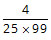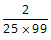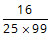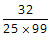# GMAT Practice Questions - Arithmetic

Must solve GMAT practice questions in arithmetic. The following topics are covered in the GMAT quant section from arithmetic:

1. Number Properties & Theory
2. Ratio and Proportions
3. Percents
4. Powers and Roots of Numbers
1. Descriptive Statistics
2. Sets
3. Counting Methods: Permutation & Combination
4. Discrete Probability

Click on the Problem Solving or the Data Sufficiency tab for practice questions. The topic from which the question has been selected and the concept tested are listed in the right column for each question. Detailed explanatory answers, solution videos and slide decks are also provided.

• 1
• If two distinct integers a and b are picked from {1, 2, 3, 4, .... 100} and multiplied, what is the probability that the resulting number has EXACTLY 3 factors? A.B.C.D.E.• Number Properties
• Discrete Probability
• Number of factors
The required probability isSolution

• 2
• Consider a set S = {2, 4, 6, 8, x, y} with distinct elements. If x and y are both prime numbers and 0 < x < 40 and 0 < y < 40, which of the following MUST be true? I. The maximum possible range of the set is greater than 33.
II. The median can never be an even number.
III. If y = 37, the average of the set will be greater than the median.

A. I only
B. I and II only
C. I and III only
D. III only
E. I, II, and III

Solution

• 3
• Three positive integers a, b, and c are such that their average is 20 and a ≤ b ≤ c. If the median is (a + 11), what is the least possible value of c? A. 23
B. 21
C. 25
D. 26
E. 24

Solution

• 1
• What is the remainder when the positive integer x is divided by 6? Statement 1: When x is divided by 7, the remainder is 5.
Statement 2: When x is divided by 9, the remainder is 3.
• Data Sufficiency
• Number Properties
• Remainders